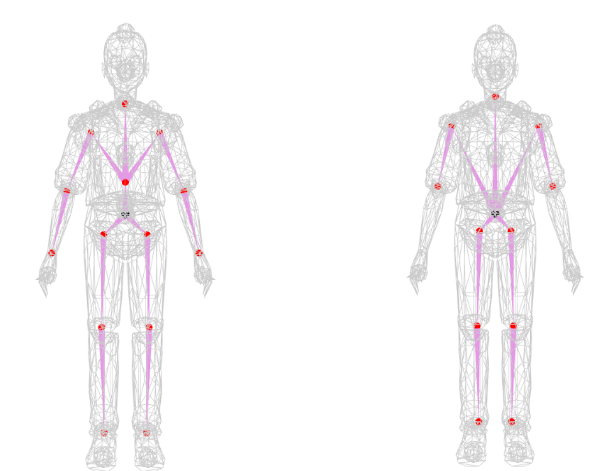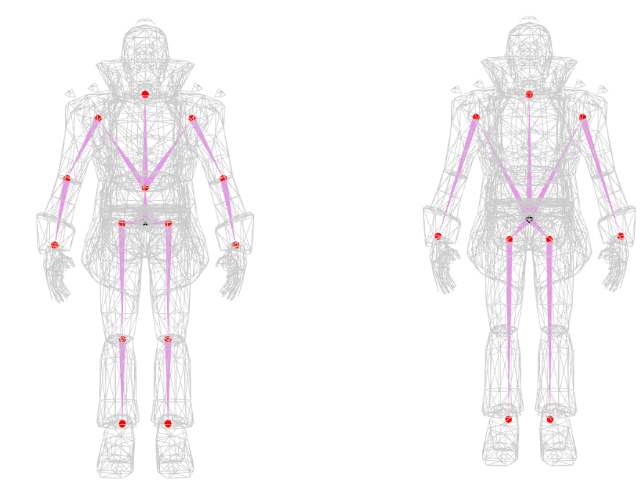# Code for SIGGRAPH 2020 paper "RigNet: Neural Rigging for Articulated Characters"zhan-xu, updated 🕥 2023-03-19 04:06:40

This is the code repository implementing the paper "RigNet: Neural Rigging for Articulated Characters" published on SIGGRAPH 2020 [Project page].

[2023.02.17] About dataset: If you are from a research lab and interested in the dataset for research-only purposes, please send a request email to me at [email protected]

[2020.11.23] There is now a great add-on for Blender based on our work, implemented by @pKrime. Please check the Github link, and the video demo.

## Dependecy and Setup

The project is developed on Ubuntu 16.04 with cuda10.0 and cudnn7.6.3. It has also been successfully tested on Windows 10. On both platforms, we suggest to use conda virtual environment.

#### For Linux user

[2021.07.20] I have tested the code on Ubuntu 18.04, with cuda 11.0. The following commands have been updated which install pytorch 1.7.1 and pytorch_geometric 1.7.2.

```conda create --name rignet_cuda11 python=3.6 conda activate rignet_cuda11```

Some necessary libraries include:

```pip install numpy scipy matplotlib tensorboard open3d==0.9.0 opencv-python pip install "rtree>=0.8,<0.9" pip install trimesh[easy] conda install pytorch==1.7.1 torchvision==0.8.2 cudatoolkit=11.0 -c pytorch pip install --no-index torch-scatter torch-sparse torch-cluster -f https://pytorch-geometric.com/whl/torch-1.7.1+cu110.html pip install torch-geometric==1.7.2```

#### For Windows user

The code has been tested on Windows 10 with cuda 10.1. The most important difference from Linux setup is, you need to download Windows-compiled Rtree from here, and install it by `pip install Rtree‑0.9.4‑cp37‑cp37m‑win_amd64.whl` (64-bit system) or `pip install Rtree‑0.9.4‑cp37‑cp37m‑win32.whl` (32-bit system). Other libraries can be installed in the same way as Linux setup instructions.

## Quick start

We provide a script for quick start. First download our trained models from here. Put the checkpoints folder into the project folder.

Check and run quick_start.py. We provide some examples in this script. Due to randomness, the results might be slightly different among each run. Generally you will get the results similar to the ones shown below:If you want to try your own models, remember to simplify the meshes so that the remeshed ones have vertices between 1K to 5K. I use quadratic edge collapse in MeshLab for this. Please name the simplified meshed as *_remesh.obj.

The predicted rigs are saved as _rig.txt. You can combine the OBJ file and _rig.txt into FBX format by running maya_save_fbx.py provided by us in Maya using mayapy. (To use numpy in mayapy, download windows compiled numpy from here and put it in mayapy library folder. For example, mine is C:\Program Files\Autodesk\Maya2019\Python\Lib\site-packages)

## Data

Our dataset ModelsResource-RigNetv1 has 2,703 models. We split it into 80% for training (2,163‬ models), 10% for validation (270 models), and 10% for testing. All models in fbx format can be downloaded here.

To use this dataset in this project, pre-processing is performed. We put the pre-processed data here, which consists of several sub-folders.

• obj: all meshes in OBJ format.
• rig_info: we store the rigging information into a txt file. Each txt file has four blocks. (1) Lines starting with "joint" define a joint with its 3D position. Each of joint line has four elements, which are joint_name, X, Y, and Z. (2) Line starting with "root" defines the name of root joint. (3) Lines starting with "hier" define the hierarchy of skeleton. Each hierarchy line has two elements, which are parent joint name and its child joint name. One parent joint can have multiple children joints. (4) Lines starting with "skin" define the skinning weights. Each skinning line follows the format as vertex_id, bind_joint_name_1, bind_weight_1, bind_joint_name_2, bind_weight_2 ... The vertex_id follows the vertice order in obj files in the above obj folder.
• obj_remesh: This folder contains the obj files of the remeshed models. Meshes with fewer than 1K vertices were subdivided, and those with more than 5K vertices were simplified; as a result all training and test meshes contained between 1K and 5K vertices.
• rig_info_remesh: Rigging information files corresponding to the remeshed obj. Joints, hierarchy and root are the same. The skinning is recalculated based on nearest neighbor from each remeshed vertex to original vertices.
• pretrain_attention: Pre-calculated supervision to pretrin the attention module, which are calculated by the script geometric_proc/compute_pretrain_attn.py. Each file is a N-by-1 text where N is the number of vertices corresponding to remeshed OBJ file, the i-th row stores the surpervision for vertex i.
• volumetric_geodesic: Pre-calculated volumetric geodesic distance between each vertex-bones pair. The algorithm is an approaximation, which is implemented in geometric_proc/compute_volumetric_geodesic.py. Each file is an N-by-B numpy array where N is the number of vertices corresponding to remeshed OBJ file, B is the number of bones, and (i, j) stores the volumetric geodesic distance between vertex i and bone j.
• vox: voxelized models used for inside/outside check. Obtained with binvox. The resolution of the grid is 88x88x88.

`python gen_dataset.py`

Remember to change the root_folder to the directory you uncompress the pre-processed data.

## Training

Notes: As new features, we have three improvements from the paper: (1) To train the joint prediction module, now we pretrain both the regression module and the attention module, and then fine-tune them together with differentiable clustering. (2) We optimized the hyper-parameters in the fine-tuning step. (3) the input feature for skinning now includes another dimension per bone (--Lf), indicating whether this bone is a virtual leaf bone or not. (To enable control from the end-joints, we presume a virtual bone for them. Please check the code for more details.)

1. Joint prediction:

1.1 Pretrain regression module: `python -u run_joint_pretrain.py --train_folder='DATASET_DIR/train/' --val_folder='DATASET_DIR/val/' --test_folder='DATASET_DIR/test/' --checkpoint='checkpoints/pretrain_jointnet' --logdir='logs/pretrain_jointnet' --train_batch=6 --test_batch=6 --lr 5e-4 --schedule 50 --arch='jointnet'`

1.2 Pretrain attention module: `python -u run_joint_pretrain.py --train_folder='DATASET_DIR/train/' --val_folder='DATASET_DIR/val/' --test_folder='DATASET_DIR/test/' --checkpoint='checkpoints/pretrain_masknet' --logdir='logs/pretrain_masknet' --train_batch=6 --test_batch=6 --lr 1e-4 --schedule 50 --arch='masknet'`

1.3 Finetune two modules with a clustering module: `python -u run_joint_finetune.py --train_folder='DATASET_DIR/train/' --val_folder='DATASET_DIR/val/' --test_folder='DATASET_DIR/test/' --checkpoint='checkpoints/gcn_meanshift' --logdir='logs/gcn_meanshift' --train_batch=1 --test_batch=1 --jointnet_lr=1e-6 --masknet_lr=1e-6 --bandwidth_lr=1e-6 --epoch=50`

2. Connectivity prediction

2.1 BoneNet: `python -u run_pair_cls.py --train_folder='DATASET_DIR/train/' --val_folder='DATASET_DIR/val/' --test_folder='DATASET_DIR/test/' --checkpoint='checkpoints/bonenet' --logdir='logs/bonenet' --train_batch=6 --test_batch=6 --lr=1e-3`

2.2 RootNet: `python -u run_root_cls.py --train_folder='DATASET_DIR/train/' --val_folder='DATASET_DIR/val/' --test_folder='DATASET_DIR/test/' --checkpoint='checkpoints/rootnet' --logdir='logs/rootnet' --train_batch=6 --test_batch=6 --lr=1e-3`

3. Skinning prediction: `python -u run_skinning.py --train_folder='DATASET_DIR/train/' --val_folder='DATASET_DIR/val/' --test_folder='DATASET_DIR/test/' --checkpoint='checkpoints/skinnet' --logdir='logs/skinnet' --train_batch=4 --test_batch=4 --lr=1e-4 --Dg --Lf`

## Issues

### The link of the dataset has been removed.

opened on 2023-04-01 12:10:19 by Kebii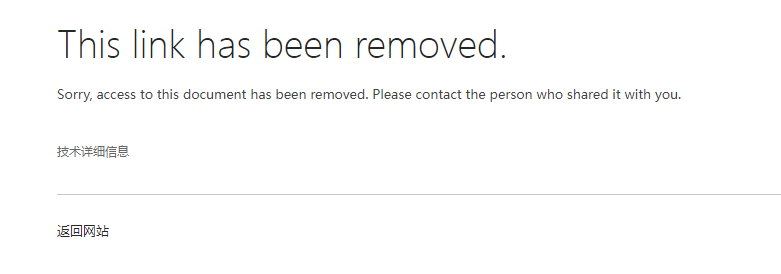How can I get the dataset? Thanks!

### Imcomplete skeleton

opened on 2023-02-28 09:26:00 by Bob-King

Hi:

we try to use the mode to generate the skeleton but have gotten an imcomplete skeleton as below image shows. Pls help have a check and let me know that's wrong, thanks. The obj file is attached in zip format.

8332_remesh.zip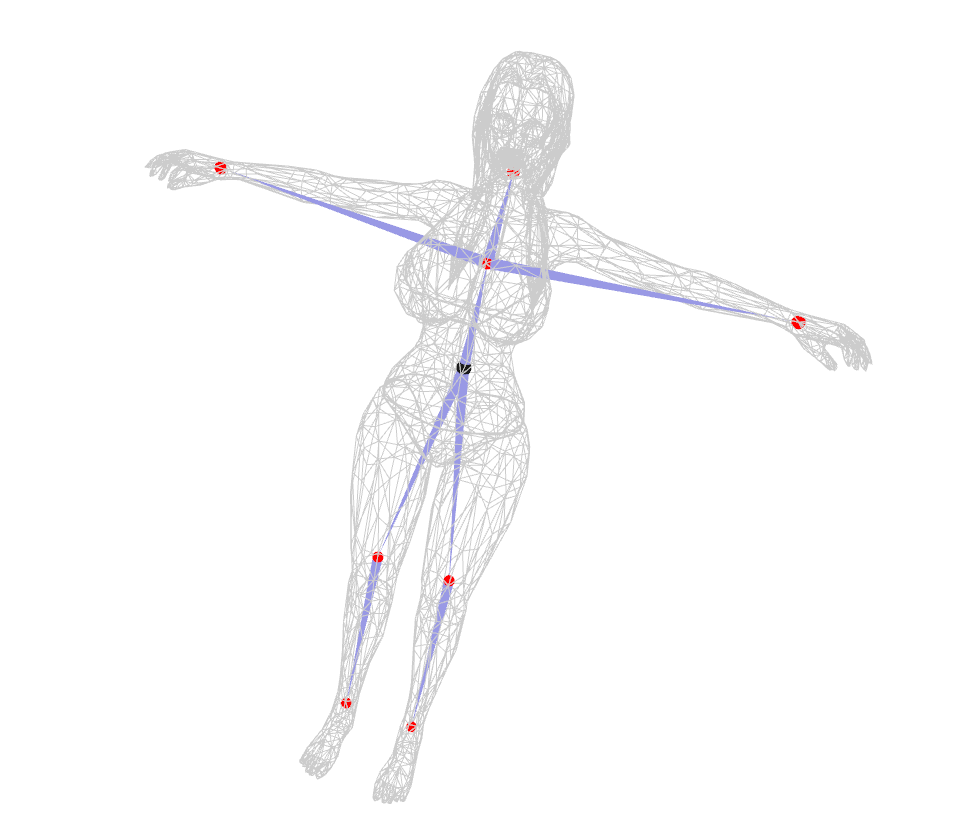### the issue on Dataset Directory variable (DATASET_DIR) for training

opened on 2023-02-27 06:38:36 by WisarutBholsithi

I thought that DATASET_DIR is referring to the directory that contain 3 text files (test_final.txt, train_final.txt, val_final.txt) along with directory that contain obj files along with the directory that contain vox files. In my case, it is ModelResource_RigNetv1_preproccessed directory inside the working directory (RigNet-master directory). Here is the way I have set up for the training which I have added the debugging code to countercheck with my working directory as wizdir/RigNet-master:

Here the command I have run and detect some issue with the directory name

`python -u run_joint_pretrain.py --train_folder='./ModelResource_RigNetv1_preproccessed/train/' --val_folder='./ModelResource_RigNetv1_preproccessed/val/' --test_folder='./ModelResource_RigNetv1_preproccessed/test/' --checkpoint='checkpoints/pretrain_jointnet' --logdir='logs/pretrain_jointnet' --train_batch=6 --test_batch=6 --lr 5e-4 --schedule 50 --arch='jointnet'`

Here is my debugging code in run_joint_pretrain.py: ``` def main(args): ... cudnn.benchmark = True print(' Total params: %.2fM' % (sum(p.numel() for p in model.parameters()) / 1000000.0)) print(args.train_folder, args.train_batch) # args.train_folder is ./ModelResource_RigNetv1_preproccessed/train/ and args.train_folder is , args.train_batch is 6 print(args.val_folder, args.test_batch) # args.train_folder is ./ModelResource_RigNetv1_preproccessed/val/ and args.train_folder is , args.train_batch is 6 print(args.test_folder, args.test_batch) # args.train_folder is ./ModelResource_RigNetv1_preproccessed/test/ and args.train_folder is , args.train_batch is 6 train_loader = DataLoader(GraphDataset(root=args.train_folder), batch_size=args.train_batch, shuffle=True, follow_batch=['joints']) # problem happened on this line val_loader = DataLoader(GraphDataset(root=args.val_folder), batch_size=args.test_batch, shuffle=False, follow_batch=['joints']) test_loader = DataLoader(GraphDataset(root=args.test_folder), batch_size=args.test_batch, shuffle=False, follow_batch=['joints']) ... if name == 'main': ... parser.add_argument('--train_folder', default='wizdir/RigNet-master/ModelResource_RigNetv1_preproccessed/train/', type=str, help='folder of training data')

``````parser.add_argument('--val_folder', default='wizdir/RigNet-master/ModelResource_RigNetv1_preproccessed/val/',
type=str, help='folder of validation data')

type=str, help='folder of testing data')
``````

... ``` Here is my Debug code in dataset/skeleton_dataset.py

``` class GraphDataset(InMemoryDataset): def init(self, root): super(GraphDataset, self).init(root) # index out of range problem self.data, self.slices = torch.load(self.processed_paths) ..... def process(self): data_list = [] i = 0.0 print(self.raw_paths, self.raw_paths[len(self.raw_paths)-1], len(self.raw_paths)) # len(self.raw_paths) is 2163 and for v_filename in self.raw_paths: print('preprecessing data complete: {:.4f}%'.format(100 * i / len(self.raw_paths))) i += 1.0 v = np.loadtxt(v_filename) # problem arise here m = np.loadtxt(v_filename.replace('_v.txt', '_attn.txt')) tpl_e = np.loadtxt(v_filename.replace('_v.txt', '_tpl_e.txt')).T geo_e = np.loadtxt(v_filename.replace('_v.txt', '_geo_e.txt')).T joints = np.loadtxt(v_filename.replace('_v.txt', '_j.txt')) adj = np.loadtxt(v_filename.replace('_v.txt', '_adj.txt'), dtype=np.uint8)

``````        vox_file = v_filename.replace('_v.txt', '.binvox')
``````

``` The result has been as shown in this screen capture: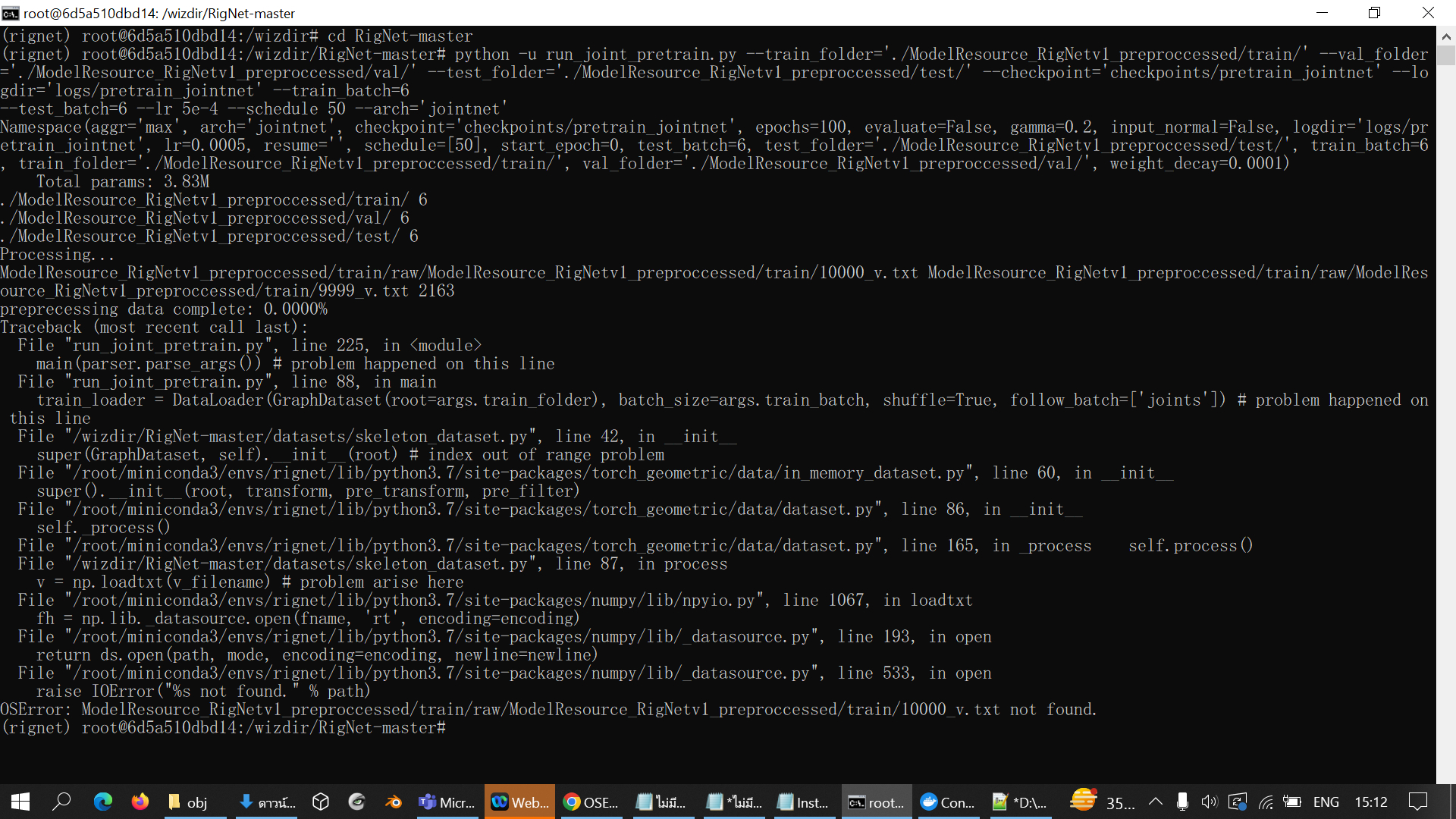However, the list variable (self.raw_paths) that show the raw path have shown the following value:

``` print(self.raw_paths, self.raw_paths[len(self.raw_paths)-1], len(self.raw_paths)) # self.raw_paths is ModelResource_RigNetv1_preproccessed/train/raw/ModelResource_RigNetv1_preproccessed/train/10000_v.txt # self.raw_paths[ len(self.raw_paths) -1] is ModelResource_RigNetv1_preproccessed/train/raw/ModelResource_RigNetv1_preproccessed/train/9999_v.txt

# len(self.raw_paths) is 2163
```

So, what to be done to make a correct directory link since file 10000_v.txt is in wizdir/RigNet-master/ModelResource_RigNetv1_preproccessed/train directory but self.raw_paths has shown the result as ModelResource_RigNetv1_preproccessed/train/raw/ModelResource_RigNetv1_preproccessed/train/10000_v.txt which is incorrect.

### Running RigNet in python3.9 and get Aborted

opened on 2022-11-25 11:55:20 by WisarutBholsithi

I have tried to install RigNet to be used in python3.9 through the installation process: 1. Installing the necessary library pip install numpy scipy matplotlib tensorboard open3d opencv-python pip install rtree pip install trimesh[easy] 2. If conda had not been installed yet, installing conda and set Evironment Variable 3. Install pytorch to the lastest version (pytorch==1.12.0) and the necessary pytorch library in the following manner: conda install pytorch==1.12.0 torchvision cudatoolkit -c pytorch pip install torch-scatter -f https://data.pyg.org/whl/torch-1.12.0+\$cu.html pip install torch-sparse -f https://data.pyg.org/whl/torch-1.12.0+\$cu.html pip install torch-cluster -f https://data.pyg.org/whl/torch-1.12.0+\$cu.html pip install torch-spline-conv -f https://data.pyg.org/whl/torch-1.12.0+\$cu.html pip install torch-geometric 4. Activate Rignet through Cuda in the following manner: conda create --name rignet_cuda11 python=3.9 conda activate rignet_cuda11

To test that everything works, from the anaconda console go into RigNet-master folder and run:

python quick_start.py

However, I got Aborted result as shown here: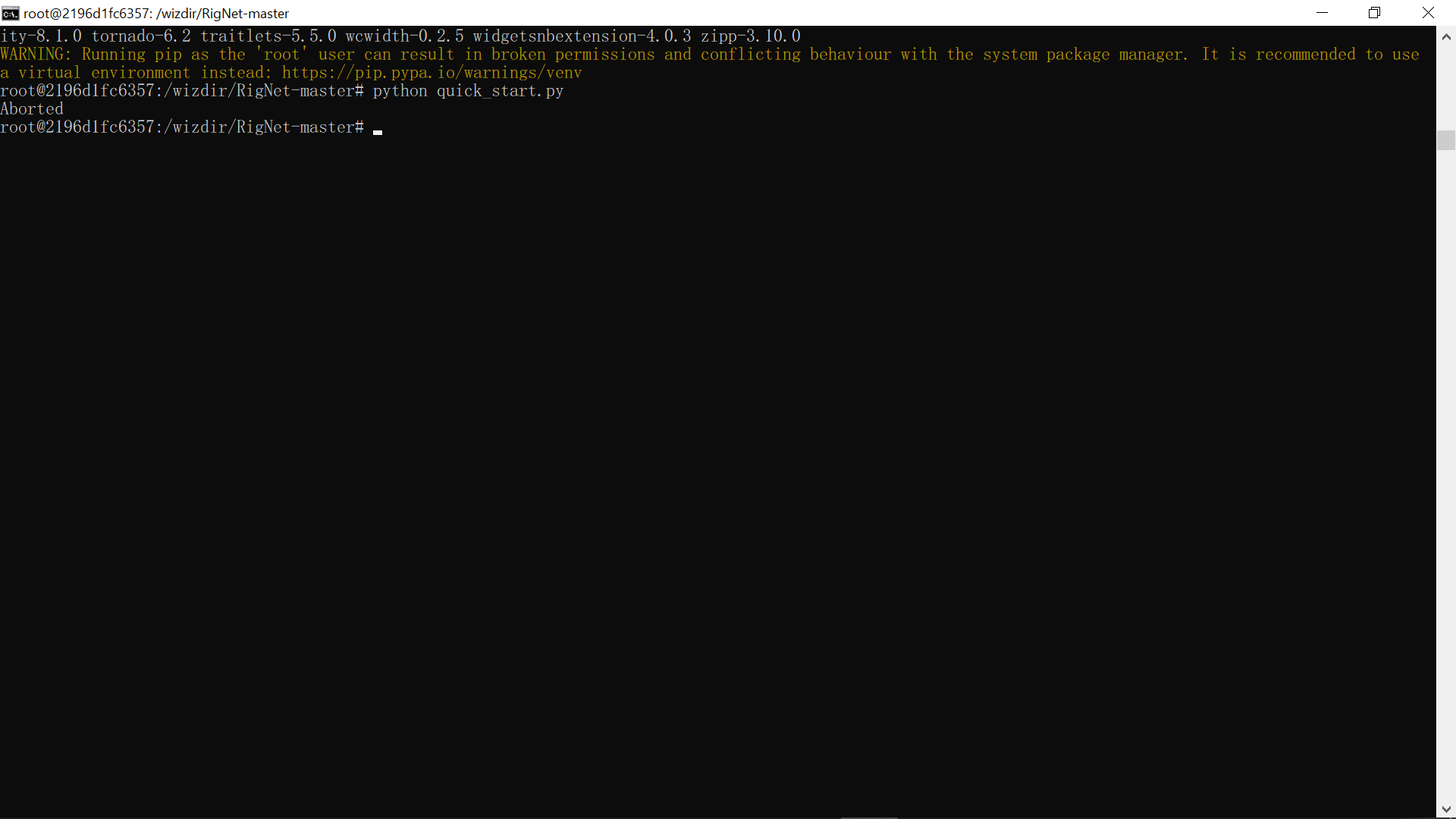Is there any solution for this problem? BTW, I still unable to access to get testing and training data from this website for training though.

### 可以提供colab版吗？

opened on 2022-11-10 12:03:49 by Asterecho

### Is it possible to generating fixed joints with certain topology?

opened on 2022-10-26 00:49:05 by siRoblox

For example I want to generate result with 14 joints with the same topology as the left one in each image, is there a suggested way to achieve it?